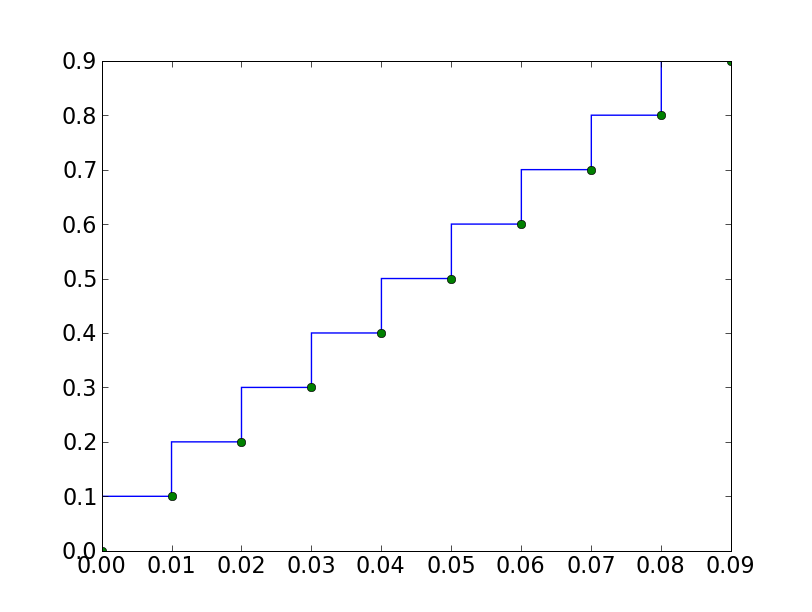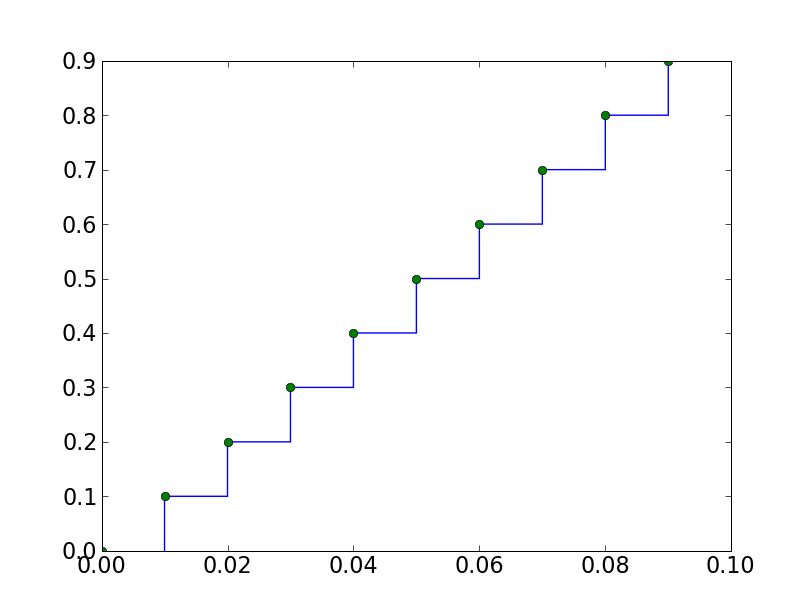# possible bug with linestyle='steps'

I think I used to use plot with linestyle=‘steps’ to plot data for zero-order hold control systems. This means that if the system is updating on a period of 0.01 seconds (100 Hz), the values should be considered held from 0 to 0.0099999999999999 and then from 0.01 to 0.01999999999 and so on each time step. So, what I want is a plot that looks like late_steps.png (hopefully attached), but what I am currently getting is early_steps.png.

This code snippet recreates my problem. My t2 hack almost makes the plot look right.

t = arange(0,0.1,0.01)
y = 10*t
clf()
plot(t,y,linestyle=‘steps’)
plot(t,y,‘o’)
savefig(‘early_steps.png’)

t2 = t+0.01
clf()
plot(t2,y,linestyle=‘steps’)
plot(t,y,‘o’)
savefig(‘late_steps.png’)

Is this a bug, is this the expected behavior for other applications, or can this be changed with some configuration setting?

Thanks,

RyanOh, and in case it matters I am running

In : matplotlib.version
Out: ‘0.98.5.2’

on Ubuntu 9.04 (with the rather lame name of Jaunty Jackolope).

···

On Fri, May 15, 2009 at 6:51 PM, Ryan Krauss <ryanlists@…287…> wrote:

I think I used to use plot with linestyle=‘steps’ to plot data for zero-order hold control systems. This means that if the system is updating on a period of 0.01 seconds (100 Hz), the values should be considered held from 0 to 0.0099999999999999 and then from 0.01 to 0.01999999999 and so on each time step. So, what I want is a plot that looks like late_steps.png (hopefully attached), but what I am currently getting is early_steps.png.

This code snippet recreates my problem. My t2 hack almost makes the plot look right.

t = arange(0,0.1,0.01)
y = 10*t
clf()
plot(t,y,linestyle=‘steps’)
plot(t,y,‘o’)
savefig(‘early_steps.png’)

t2 = t+0.01
clf()
plot(t2,y,linestyle=‘steps’)
plot(t,y,‘o’)
savefig(‘late_steps.png’)

Is this a bug, is this the expected behavior for other applications, or can this be changed with some configuration setting?

Thanks,

Ryan

RTFM:

plot(t,y, drawstyle=‘steps-post’)

http://matplotlib.sourceforge.net/examples/pylab_examples/set_and_get.html

especially

``````>>> line, = plot([1,2,3])
>>> setp(line, linestyle='--')

``````
···

On Fri, May 15, 2009 at 6:52 PM, Ryan Krauss <ryanlists@…287…> wrote:

Oh, and in case it matters I am running

In : matplotlib.version
Out: ‘0.98.5.2’

on Ubuntu 9.04 (with the rather lame name of Jaunty Jackolope).

On Fri, May 15, 2009 at 6:51 PM, Ryan Krauss <ryanlists@…287…> wrote:

I think I used to use plot with linestyle=‘steps’ to plot data for zero-order hold control systems. This means that if the system is updating on a period of 0.01 seconds (100 Hz), the values should be considered held from 0 to 0.0099999999999999 and then from 0.01 to 0.01999999999 and so on each time step. So, what I want is a plot that looks like late_steps.png (hopefully attached), but what I am currently getting is early_steps.png.

This code snippet recreates my problem. My t2 hack almost makes the plot look right.

t = arange(0,0.1,0.01)
y = 10*t
clf()
plot(t,y,linestyle=‘steps’)
plot(t,y,‘o’)
savefig(‘early_steps.png’)

t2 = t+0.01
clf()
plot(t2,y,linestyle=‘steps’)
plot(t,y,‘o’)
savefig(‘late_steps.png’)

Is this a bug, is this the expected behavior for other applications, or can this be changed with some configuration setting?

Thanks,

Ryan

Ryan Krauss-2 wrote:

RTFM:

plot(t,y, drawstyle='steps-post')

Actually, 'steps-pre' (which is the default) and 'steps-post' seem to have
swapped definitions.
Here is what the docs say:
*where*: [ 'pre' | 'post' | 'mid' ]
If 'pre', the interval from x[i] to x[i+1] has level y[i]
If 'post', that interval has level y[i+1]
If 'mid', the jumps in *y* occur half-way between the
*x*-values.

In fact both the default behavior and what you get with steps-pre are what
SHOULD happen with steps-post. And steps-post (as you point out) does what
should be the default behavior and that of steps-pre.

I have filed a bug report on this, since it is very important that this work
as expected. As the original poster pointed out, this used to work
correctly but recently seems to have gotten broken.

Cheers,
-- Paul

···

--
View this message in context: http://www.nabble.com/possible-bug-with-linestyle%3D'steps'-tp23568959p24542440.html
Sent from the matplotlib - users mailing list archive at Nabble.com.

I am looking first at the behavior of plot with the drawstyle property
set -- let's make sure this is correct before turning to the steps
command, which just uses plot with the drawstyle set -- here is my
test code

import numpy as np
import matplotlib.pyplot as plt

fig = plt.figure()

a = np.array([1,2,3,4,5])

styles = 'default' , 'steps' , 'steps-pre' , 'steps-mid' , 'steps-post'
styles = 'steps' , 'steps-pre'
for ls in styles:
ax.plot(a, ls=ls, label=ls, lw=2)

ax.legend(loc='upper left')

plt.show()

pre causes the step to rise on the x[i], post causes it to rise on
x[i+1] and mid in the middle. This seems like the correct behavior.
So it does look like the docstring for 'step' is incorrect, and I've

*where*: [ 'pre' | 'post' | 'mid' ]
If 'pre', the interval from x[i] to x[i+1] has level y[i+1]

If 'post', that interval has level y[i]

If 'mid', the jumps in *y* occur half-way between the

JDH

···

On Fri, Jul 17, 2009 at 5:15 PM, Paul Ray<Paul.Ray@...706...> wrote:

Ryan Krauss-2 wrote:

RTFM:

plot(t,y, drawstyle='steps-post')

Actually, 'steps-pre' (which is the default) and 'steps-post' seem to have
swapped definitions.
Here is what the docs say:
*where*: [ 'pre' | 'post' | 'mid' ]
If 'pre', the interval from x[i] to x[i+1] has level y[i]
If 'post', that interval has level y[i+1]
If 'mid', the jumps in *y* occur half-way between the
*x*-values.

In fact both the default behavior and what you get with steps-pre are what
SHOULD happen with steps-post. And steps-post (as you point out) does what
should be the default behavior and that of steps-pre.

I have filed a bug report on this, since it is very important that this work
as expected. As the original poster pointed out, this used to work
correctly but recently seems to have gotten broken.

Hi,

I just installed matplotlib 0.99.0 and I see that this problem is still there.
The command plot(a,ls='steps') is equivalent to plot(a,ls='steps-pre') and both cause the first value of the array to NOT be plotted. This is REALLY not what should happen when one plots an array with several values. It is fine if there is a custom option to make that behavior (like 'steps-pre'), but the default for ls='steps' or step(x,y) must be to have the first flat level at the level of the first entry (what the 'steps-post' does).

The docstring now correctly describes the behavior, so that is good, but please please make the default "steps" be "steps-post".

Thanks,

-- Paul

···

On Jul 19, 2009, at 9:35 AM, John Hunter wrote:

On Fri, Jul 17, 2009 at 5:15 PM, Paul Ray<Paul.Ray@...706...> > wrote:

Ryan Krauss-2 wrote:

RTFM:

plot(t,y, drawstyle='steps-post')

Actually, 'steps-pre' (which is the default) and 'steps-post' seem to have
swapped definitions.
Here is what the docs say:
*where*: [ 'pre' | 'post' | 'mid' ]
If 'pre', the interval from x[i] to x[i+1] has level y[i]
If 'post', that interval has level y[i+1]
If 'mid', the jumps in *y* occur half-way between the
*x*-values.

In fact both the default behavior and what you get with steps-pre are what
SHOULD happen with steps-post. And steps-post (as you point out) does what
should be the default behavior and that of steps-pre.

I have filed a bug report on this, since it is very important that this work
as expected. As the original poster pointed out, this used to work
correctly but recently seems to have gotten broken.

I am looking first at the behavior of plot with the drawstyle property
set -- let's make sure this is correct before turning to the steps
command, which just uses plot with the drawstyle set -- here is my
test code

import numpy as np
import matplotlib.pyplot as plt

fig = plt.figure()

a = np.array([1,2,3,4,5])

styles = 'default' , 'steps' , 'steps-pre' , 'steps-mid' , 'steps-post'
styles = 'steps' , 'steps-pre'
for ls in styles:
ax.plot(a, ls=ls, label=ls, lw=2)

ax.legend(loc='upper left')

plt.show()

pre causes the step to rise on the x[i], post causes it to rise on
x[i+1] and mid in the middle. This seems like the correct behavior.
So it does look like the docstring for 'step' is incorrect, and I've